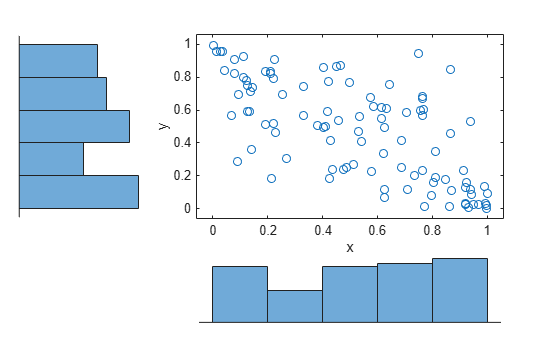Main Content

# copulaparam

Copula parameters as function of rank correlation

## Syntax

``rho = copulaparam('Gaussian',r)``
``rho = copulaparam('t',r,nu)``
``alpha = copulaparam(family,r)``
``___ = copulaparam(___,Name,Value)``

## Description

example

````rho = copulaparam('Gaussian',r)` returns the linear correlation parameters, `rho`, that correspond to a Gaussian copula with Kendall’s rank correlation, `r`.```
````rho = copulaparam('t',r,nu)` returns the linear correlation parameters, `rho`, that correspond to a t copula with Kendall’s rank correlation, `r`, and degrees of freedom, `nu`.```
````alpha = copulaparam(family,r)` returns the copula parameter, `alpha`, that corresponds to a bivariate Archimedean copula of the type specified by `family`, with Kendall’s rank correlation, `r`.```
````___ = copulaparam(___,Name,Value)` returns the correlation parameter using any of the previous syntaxes, with additional options specified by one or more `Name,Value` pair arguments. For example, you can specify whether the input rank correlation value is Spearman’s rho or Kendall’s tau.```

## Examples

collapse all

Generate correlated random data from a beta distribution using a bivariate Gaussian copula with Kendall's tau rank correlation equal to -0.5.

Compute the linear correlation parameter from the rank correlation value.

```rng default % For reproducibility tau = -0.5; rho = copulaparam('Gaussian',tau)```
```rho = -0.7071 ```

Use a Gaussian copula to generate a two-column matrix of dependent random values.

`u = copularnd('gaussian',rho,100);`

Each column contains 100 random values between 0 and 1, inclusive, sampled from a continuous uniform distribution.

Create a `scatterhist` plot to visualize the random numbers generated using the copula.

```figure scatterhist(u(:,1),u(:,2))```The histograms show that the data in each column of the copula has a marginal uniform distribution. The scatterplot shows that the data in the two columns is negatively correlated.

Use the inverse cdf function `betainv` to transform each column of the uniform marginal distributions into random numbers from a beta distribution. In the first column, the first shape parameter A is equal to 1, and a second shape parameter B is equal to 2. In the second column, the first shape parameter A is equal to 1.5, and a second shape parameter B is equal to 2.

`b = [betainv(u(:,1),1,2), betainv(u(:,2),1.5,2)];`

Create a `scatterhist` plot to visualize the correlated beta distribution data.

```figure scatterhist(b(:,1),b(:,2))```The histograms show the marginal beta distributions for each variable. The scatterplot shows the negative correlation.

Verify that the sample has a rank correlation approximately equal to the initial value for Kendall's tau.

`tau_sample = corr(b,'type','kendall')`
```tau_sample = 2×2 1.0000 -0.5135 -0.5135 1.0000 ```

The sample rank correlation of -0.5135 is approximately equal to the -0.5 initial value for tau.

## Input Arguments

collapse all

Copula rank correlation, returned as a scalar value or matrix of scalar values.

• If `r` is a scalar correlation coefficient, then `rho` is a scalar correlation coefficient corresponding to a bivariate copula.

• If `r` is a p-by-p correlation matrix, then `rho` is a p-by-p correlation matrix.

If the copula is specified as one of the bivariate Archimedean copula types (`'Clayton'`, `'Frank'`, or `'Gumbel'`), then `r` is a scalar value.

Degrees of freedom for the t copula, specified as a positive integer value.

Data Types: `single` | `double`

Bivariate Archimedean copula family, specified as one of the following.

 `'Clayton'` Clayton copula `'Frank'` Frank copula `'Gumbel'` Gumbel copula

### Name-Value Pair Arguments

Specify optional comma-separated pairs of `Name,Value` arguments. `Name` is the argument name and `Value` is the corresponding value. `Name` must appear inside quotes. You can specify several name and value pair arguments in any order as `Name1,Value1,...,NameN,ValueN`.

Example: `'type','Spearman'` computes Spearman’s rank correlation.

Type of rank correlation, specified as the comma-separated pair consisting of `'type'` and one of the following.

• `'Kendall'` — Indicates that the input value for `r` is a Kendall’s tau correlation value

• `'Spearman'` — Indicates that the input value for `r` is a Spearman’s rho rank correlation value

`copulaparam` uses an approximation to Spearman’s rank correlation for copula families that do not have an existing analytic formula. The approximation is based on a smooth fit to values computed at discrete values of the copula parameters. For a t copula, the approximation is accurate for degrees of freedom larger than 0.05.

Example: `'type','Spearman'`

## Output Arguments

collapse all

Linear correlation parameter, returned as a scalar value or matrix of scalar values.

• If `r` is a scalar correlation coefficient, then `rho` is a scalar correlation coefficient corresponding to a bivariate copula.

• If `r` is a p-by-p correlation matrix, then `rho` is a p-by-p correlation matrix.

Bivariate Archimedean copula parameter, returned as a scalar value. Permitted values for `alpha` depend on the specified copula family.

Copula FamilyPermitted Alpha Values
`'Clayton'`[0,∞)
`'Frank'`(-∞,∞)
`'Gumbel'`[1,∞)

Data Types: `single` | `double`

## See Also

### Topics

Introduced in R2006a

Download ebook